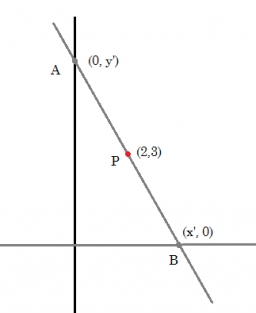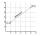# Distance between 2 points

Find the distance between the points (7, -9), (-1, -9)

Correct result:

d =  8

#### Solution:We would be pleased if you find an error in the word problem, spelling mistakes, or inaccuracies and send it to us. Thank you!Tips to related online calculators
For Basic calculations in analytic geometry is helpful line slope calculator. From coordinates of two points in the plane it calculate slope, normal and parametric line equation(s), slope, directional angle, direction vector, the length of segment, intersections the coordinate axes etc.
Do you want to convert length units?
Pythagorean theorem is the base for the right triangle calculator.

#### You need to know the following knowledge to solve this word math problem:

We encourage you to watch this tutorial video on this math problem:

## Next similar math problems:

• Here isHere is a data set (n=117) that has been sorted. 10.4 12.2 14.3 15.3 17.1 17.8 18 18.6 19.1 19.9 19.9 20.3 20.6 20.7 20.7 21.2 21.3 22 22.1 22.3 22.8 23 23 23.1 23.5 24.1 24.1 24.4 24.5 24.8 24.9 25.4 25.4 25.5 25.7 25.9 26 26.1 26.2 26.7 26.8 27.5 27.6 2
• Vertices of a right triangleShow that the points D(2,1), E(4,0), F(5,7) are vertices of a right triangle.
• DistanceCalculate distance between two points X[18; 19] and W[20; 3].
• Three pointsThree points A (-3;-5) B (9;-10) and C (2;k) . AB=AC What is value of k?
• Find the 3Find the distance and mid-point between A(1,2) and B(5,5).
• Speed of Slovakian trainsRudolf decided to take the train from the station 'Ostratice' to 'Horné Ozorovce'. In the train timetables found train Os 5409 : km 0 Chynorany 15:17 5 Ostratice 15:23 15:23 8 Rybany 15:27 15:27 10 Dolné Naštice 15:31 15:31 14 Bánovce nad Bebravou 15:35 1
• The fenceI'm building a fence. Late is rounded up in semicircle. The tops of late in the field between the columns are to copy an imaginary circle. The tip of the first and last lath in the field is a circle whose radius is unknown. The length of the circle chord
• CirclesIn the circle with a radius 7.5 cm are constructed two parallel chord whose lengths are 9 cm and 12 cm. Calculate the distance of these chords (if there are two possible solutions write both).
• Distance problemA=(x, x) B=(1,4) Distance AB=√5, find x;
• Center of line segmentCalculate the distance of the point X [1,3] from the center of the line segment x = 2-6t, y = 1-4t ; t is .
• Calculate 6Calculate the distance of a point A[0, 2] from a line passing through points B[9, 5] and C[1, -1].
• ForcesIn point O acts three orthogonal forces: F1 = 20 N, F2 = 7 N and F3 = 19 N. Determine the resultant of F and the angles between F and forces F1, F2 and F3.
• DistanceWha is the distance between the origin and the point (18; 22)?
• Right angled triangle 2LMN is a right angled triangle with vertices at L(1,3), M(3,5) and N(6,n). Given angle LMN is 90° find n
• MO - trianglesOn the AB and AC sides of the triangle ABC lies successive points E and F, on segment EF lie point D. The EF and BC lines are parallel and is true this ratio FD:DE = AE:EB = 2:1. The area of ABC triangle is 27 hectares and line segments EF, AD, and DB seg
• Two chordsIn a circle with radius r = 26 cm two parallel chords are drawn. One chord has a length t1 = 48 cm and the second has a length t2 = 20 cm, with the center lying between them. Calculate the distance of two chords.
• Triangle IRTIn isosceles right triangle ABC with right angle at vertex C is coordinates: A (-1, 2); C (-5, -2) Calculate the length of segment AB.